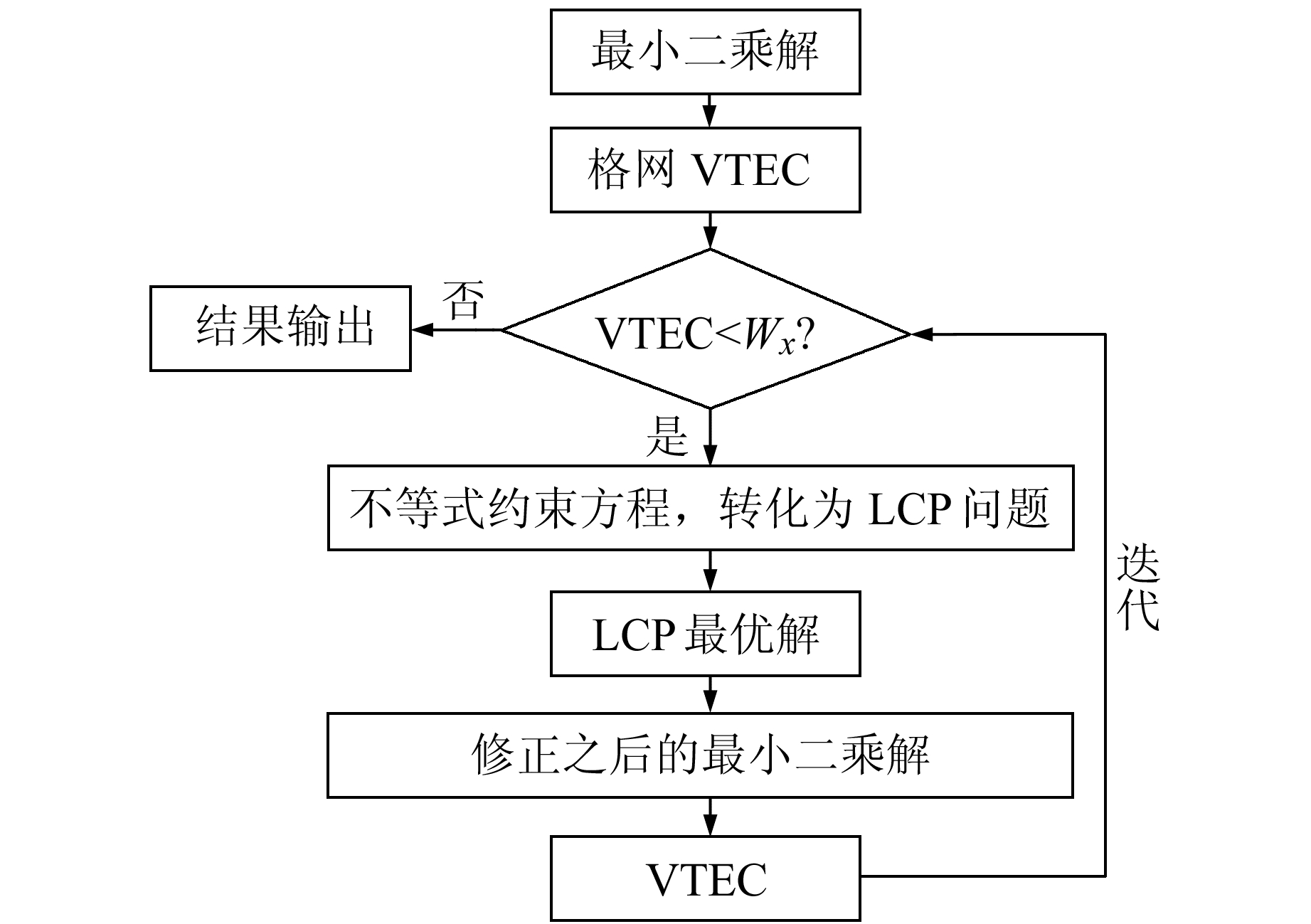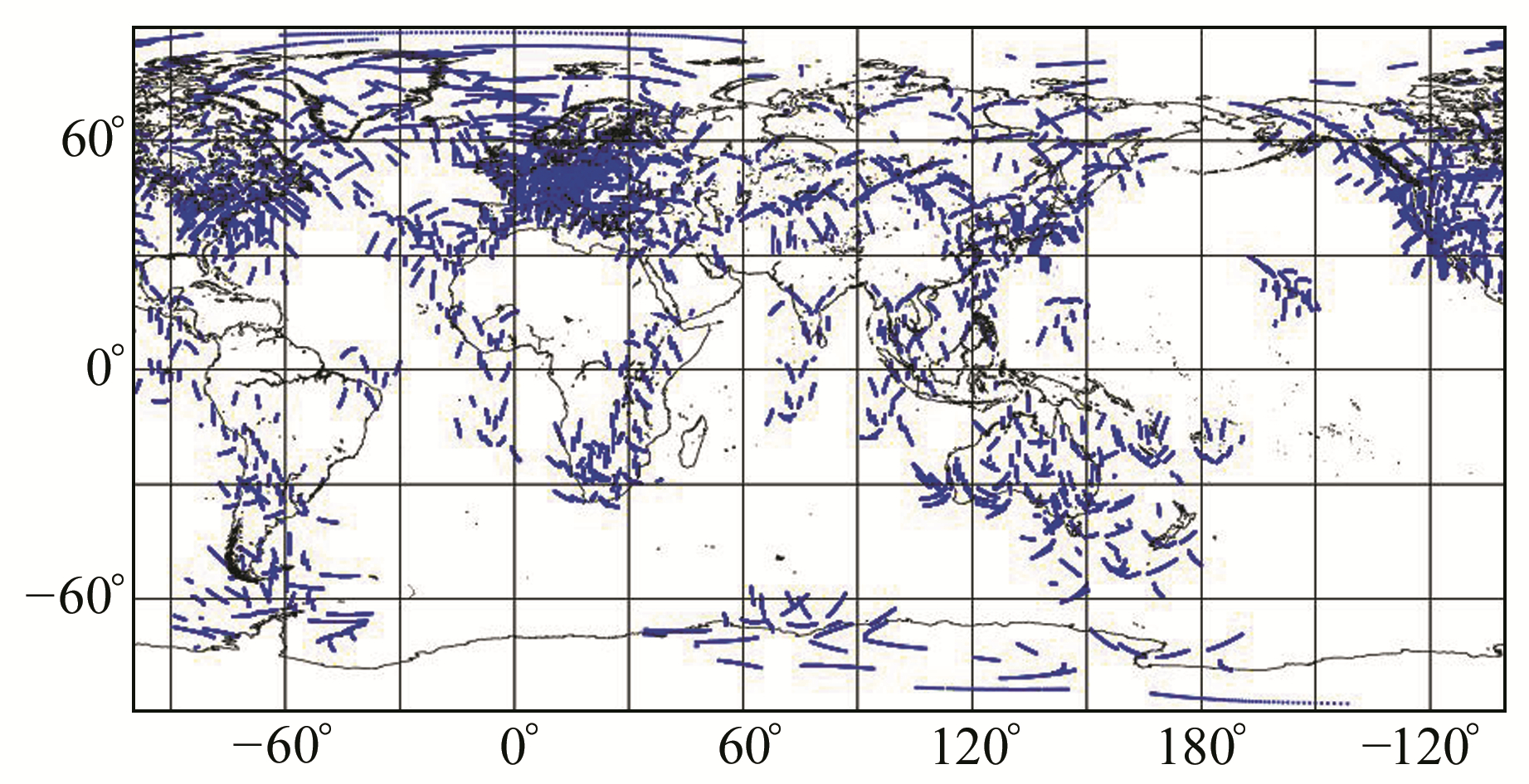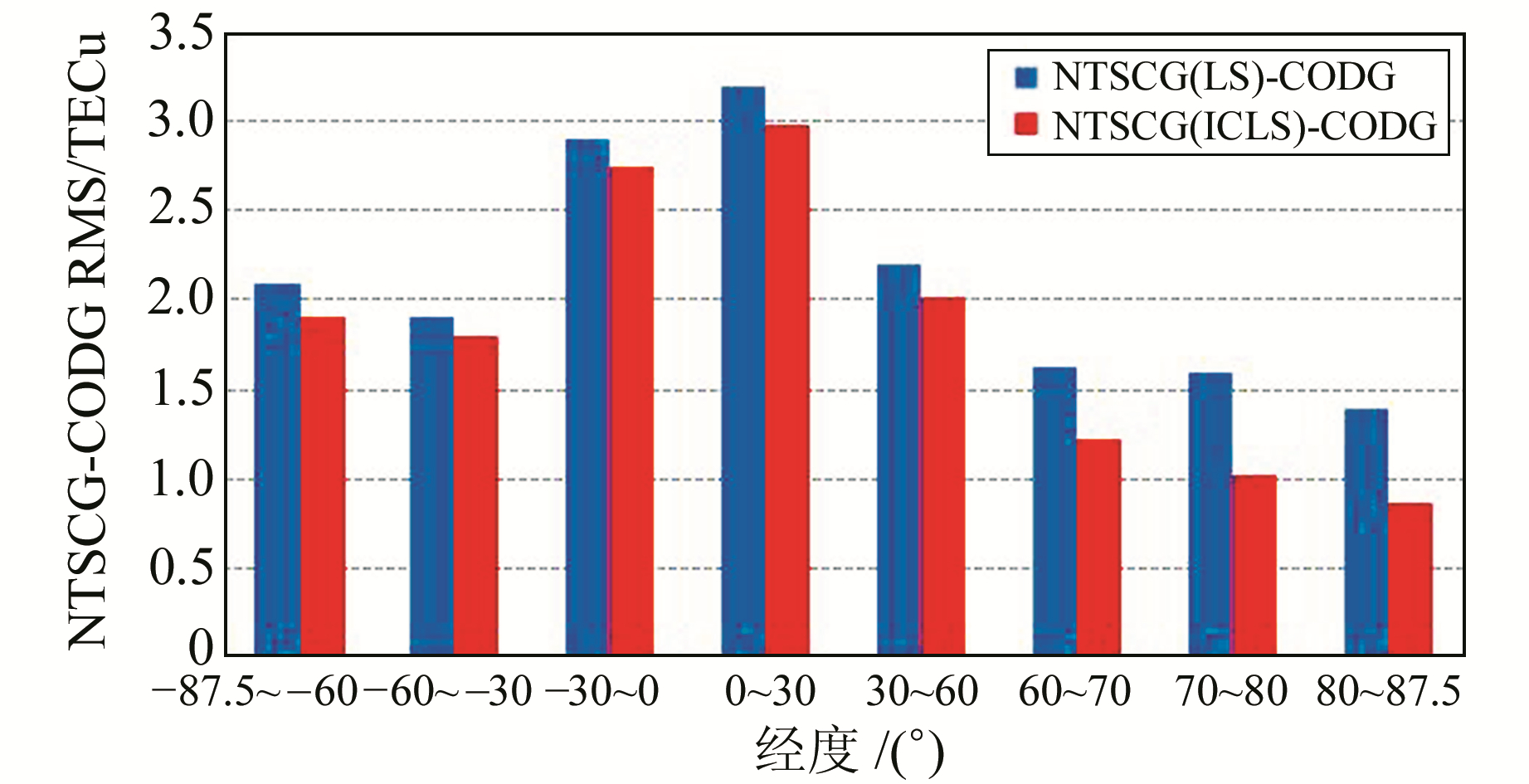﻿ 基于不等式约束算法改善高纬度地区GNSS电离层建模精度文章快速检索 高级检索
 大地测量与地球动力学2019, Vol. 39Issue (7): 722-727  DOI: 10.14075/j.jgg.2019.07.011引用本文HUANG Xiaodong, TU Rui, LIU Jinhai, et al. Inequality Constraints Algorithm to Improve the High Latitudes GNSS Ionosphere Modeling Accuracy[J]. Journal of Geodesy and Geodynamics, 2019, 39(7): 722-727.Foundation support

National Key Research and Development Program of China, No.2016YFB0501804; Open Fund for State Key Laboratories of Geo-Information Engineering, No.SKLGIE2016-M-2-4; National Natural Science Foundation of China, No.41504006, 41674034;One Hundred Person Project of CAS, No.Y620YC1701;Key Research and Development Program of Frontier Science and Technology, No.QYZDB-SSW-DQC028.

About the first author

HUANG Xiaodong, postgraduate, majors in ionosphere modeling based on GNSS, E-mail:ntschxd@163.com.

文章历史

1. 中国科学院国家授时中心，西安市书院东路3号，710600;
2. 中国科学院大学，北京市玉泉路19号(甲)，100049;
3. 中国科学院精密导航定位与定时技术重点实验室，西安市书院东路3号，710600;
4. 地理信息工程国家重点实验室，西安市雁塔路中段1号，710054

1 基于GPS数据建立全球VTEC模型

 $\begin{array}{*{20}{c}} {\tilde P_{2j}^i - \tilde P_{1j}^i = 40.28 \times \frac{{{\rm{TEC}}}}{{f_2^2}} - }\\ {40.28 \times \frac{{{\rm{TEC}}}}{{f_1^2}} + {\rm{d}}{q_j} + {\rm{d}}{q^i}} \end{array}$ (1)

2 附不等式约束的最小二乘算法电离层建模

 $\begin{array}{*{20}{c}} {\sum\limits_{n = 0}^{{n_{\max }}} {\sum\limits_{m = 0}^n {{{\tilde P}_{nm}}\left( {\sin \varphi } \right)\left( {{{\tilde A}_{nm}}\cos \left( {m\mu } \right) + } \right.} } }\\ {\left. {{{\tilde B}_{nm}}\sin \left( {m\mu } \right)} \right) \ge {W_x}} \end{array}$ (5)

 $\left\{ \begin{array}{l} \mathit{\boldsymbol{E}} = \mathit{\boldsymbol{G}}{\mathit{\boldsymbol{N}}^{ - 1}}{\mathit{\boldsymbol{G}}^{\rm{T}}}\\ \mathit{\boldsymbol{c}} = \mathit{\boldsymbol{G}}{{\mathit{\boldsymbol{\hat X}}}_0} - \mathit{\boldsymbol{W}} \end{array} \right.$ (6)

 $\left\{ \begin{array}{l} \mathit{\boldsymbol{h}} = \mathit{\boldsymbol{E\mu }} + \mathit{\boldsymbol{c}}\\ {\mathit{\boldsymbol{\mu }}^{\rm{T}}}\mathit{\boldsymbol{h}} = 0,\mu ,h \ge 0 \end{array} \right.$ (7)

 ${\mathit{\boldsymbol{\hat X}}_{{\rm{ICLS}}}} = {\mathit{\boldsymbol{\hat X}}_{\rm{0}}} + {\mathit{\boldsymbol{N}}^{ - 1}}{\mathit{\boldsymbol{G}}^{\rm{T}}}\mathit{\boldsymbol{\hat \mu }}$ (8)图 1 不等式约束最小二乘算法电离层建模流程 Fig. 1 Flow chart of ICLS ionospheric modeling
3 实验及结果分析图 2 2018-01-05 UT11：30~12：00穿刺点分布 Fig. 2 IPP distribution during UT 11:30-12:00 on Jan.5 2018

 $\sum\limits_{i = 1}^N {{\rm{DC}}{{\rm{B}}^i}} = 0$ (9)表 1 数据及模型选取策略 Tab. 1 Data and model select strategies图 3 2018-01-05 UT12：00 NTSCG(LS)全球VTEC分布 Fig. 3 Global VTEC distribution of NTSCG(LS) at UT12:00 on Jan.5 2018图 4 2018-01-05 UT12：00 NTSCG(ICLS)全球VTEC分布 Fig. 4 Global VTEC distribution of NTSCG(ICLS) at 12:00 on Jan.5 2018图 5 2018-01-05 UT12:00 NTSCG(LS)与CODG全球VTEC差异分布 Fig. 5 Global VTEC of NTSCG(LS)-CODG residual map at UT12:00 on Jan.5 2018图 6 2018-01-05 UT12:00 NTSCG(ICLS)与CODG全球VTEC差异分布 Fig. 6 Global VTEC of NTSCG(ICLS)-CODG residual map at UT12:00 on Jan.5 2018

 $\left\{ \begin{array}{l} {\rm{mean}} = \frac{1}{N}\sum\limits_{i = 1}^N {\left( {{\rm{VTE}}{{\rm{C}}_k} - {\rm{VTE}}{{\rm{C}}_{{\rm{CODE}}}}} \right)} \\ {\rm{RMS}} = \sqrt {\frac{{\sum\limits_{i = 1}^N {{{\left( {{\rm{VTE}}{{\rm{C}}_k} - {\rm{VTE}}{{\rm{C}}_{{\rm{CODE}}}}} \right)}^2}} }}{N}} \end{array} \right.$ (10)表 2 NTSCG和CODG一致性分析 Tab. 2 Consistency analysis of NTSCG and CODG图 7 NTSCG与CODG之间全球TEC差异RMS按纬度统计结果 Fig. 7 Results of global TEC residual of NTSCG and CODG based on latitude

4 结语

  李征航, 张小红. 卫星导航定位新技术及高精度数据处理方法[M]. 武汉: 武汉大学出版社, 2009 (Li Zhenghang, Zhang Xiaohong. New Technology of Satellite Navigation and Positioning and High Precision Data Processing Method[M]. Wuhan: Wuhan University Press, 2009) (0)  中国卫星导航系统管理办公室.北斗卫星导航系统空间信号接口控制文件(1.0)[Z].北京, 2012 (China Satellite Navagation System Management Office.Beidou Satellite Navagation System Spatial Signal Interface Control File 1.0[Z]. Beijing, 2012) (0)  韩文慧.不等式约束算法在地基GNSS全球电离层延迟建模中的应用[D].武汉: 武汉大学, 2012 (Han Wenhui. The Application of Inequality Constraints Least Squares in Global Ionospheric Mapping with IGS Ground based GNSS Network[D]. Wuhan: Wuhan University, 2012) (0)  Remodi B W. Real-Time Centimeter-Accurary GPS:Initial While in Motion (Warm Startversus Cold Start)[J]. Journal of the Institute of Navigation, 1993, 40(2): 199-208 DOI:10.1002/navi.1993.40.issue-2 (0)  Zhu J, Santerre R, Chang X W. A Bayesian for Linear, Inequality-Constrained Adjustment and Its Application to GPS Positioning[J]. Journal of Geodesy, 2005, 78: 528-524 DOI:10.1007/s00190-004-0425-y (0)  聂文峰, 胡伍生. 利用GPS双频数据进行区域电离层TEC提取[J]. 武汉大学学报:信息科学版, 2014, 39(9): 1 022-1 027 (Nie Wenfeng, Hu Wusheng. Extraction of Regional Ionospheric TEC from GPS Dual Observation[J]. Geomatics and Information Science of Wuhan University, 2014, 39(9): 1 022-1 027) (0)  龚阳昭, 蔡昌盛. 一种利用北斗GNSS三频观测值计算绝对电离层TEC的方法[J]. 大地测量与地球动力学, 2014, 37(2): 205-208 (Gong Yangzhao, Cai Changsheng. A Calculation Method of Ionospheric TEC Using Triple-Frequency GNSS Observation[J]. Journal of Geodesy and Geodynamics, 2014, 37(2): 205-208) (0)  袁运斌.基于GPS的电离层监测及其延迟改正理论与方法的研究[D].武汉: 中国科学院测量与地球物理研究所, 2002 (Yuan Yunbin. Study on Theories and Methods of Correcting Ionospheric Delay and Monitoring Ionosphere Based on GPS[D]. Wuhan: Institute of Geodesy and Geophysics, CAS, 2002) http://cdmd.cnki.com.cn/Article/CDMD-80057-2003040660.htm (0)  宋迎春, 朱建军, 罗德仁, 等. 附非负约束平差模型的最小二乘估计[J]. 武汉大学学报:信息科学版, 2008, 33(9): 907-909 (Song Yingchun, Zhu Jianjun, Luo Deren, et al. Least-Squares Estimation of Nonnegative Constrained Adjustment Model[J]. Geomatics and Information Science of Wuhan University, 2008, 33(9): 907-909) (0)  章红平.基于地基GPS的中国区域电离层监测与延迟改正研究[D].上海: 中国科学院上海天文台, 2006 (Zhang Hongping. Study on Regional Ionospheric Monitoring and Delay Correction in China Based on Ground-Based GPS[D]. Shanghai: Shanghai Astronomical Observatory, CAS, 2006) http://cdmd.cnki.com.cn/Article/CDMD-80022-2006109504.htm (0)  任晓东.多系统GNSS电离层TEC高精度建模及差分码偏差精确估计[D].武汉: 武汉大学, 2017 (Ren Xiaodong. Theory and Methodology of Ionospheric TEC Modeling and Differential Code Biases Estimation with Multi-GNSS[D]. Wuhan: Wuhan University, 2017) (0)
Inequality Constraints Algorithm to Improve the High Latitudes GNSS Ionosphere Modeling Accuracy
HUANG Xiaodong1,2,3TU Rui1,2,3,4     LIU Jinhai1,2,3     ZHANG Rui1,3     LU Xiaochun1,3
1. National Time Service Center, CAS, 3 East-Shuyuan Road, Xi'an 710600, China;
2. University of Chinese Academy of Sciences, A19 Yuquan Road, Beijing 100049, China;
3. Key Laboratory of Precision Navigation and Timing Technology, CAS, 3 East-Shuyuan Road, Xi'an 710600, China;
4. State Key Laboratory of Geo-Information Engineering, 1 Mid-Yanta Road, Xi'an 710054, China
Abstract: Due to uneven distribution of the international GNSS service (IGS) organization tracking stations in high latitudes, the observation data used for ionospheric fitting modeling are uneven and incomplete, leading to inadequate modeling accuracy of the total ionospheric content in this region. When the ionospheric fit model is established using observation data from these IGS tracking stations, the grid output values of the ionospheric fitting model contain a large number of negative and zero values, which are contrary to the actual physical meaning of the ionosphere. Aiming at this problem, we adopt the ionospheric grid output negative point, zero point to join inequality constraint conditions, and the inequality constraint least square method, to calculate parameters of optimization. Experimental results show that the algorithm shows a significant improvement in reducing the large number of zeros and negative values in the sparse high-latitude areas, and modeling accuracy of the global ionosphere model is also improved.
Key words: IGS; global ionosphere model; TEC; high-latitude areas; inequality constraint